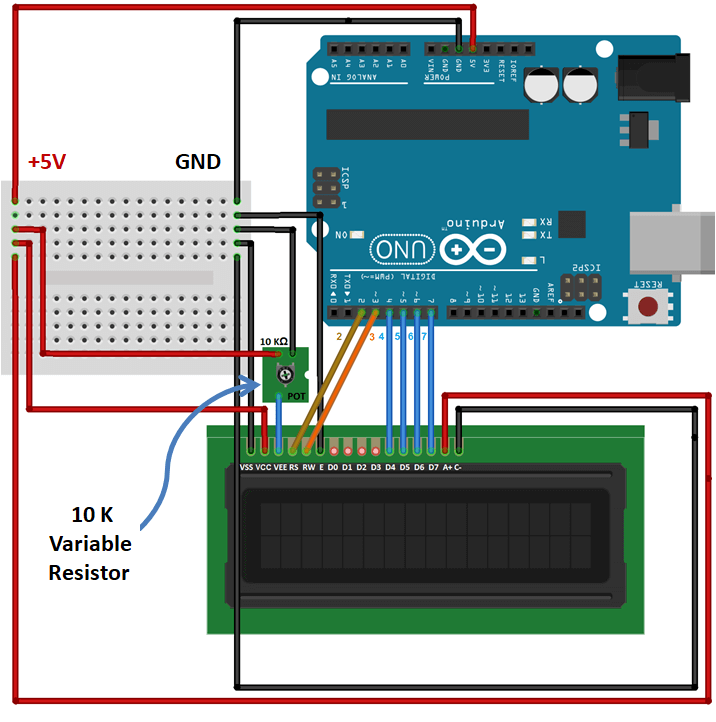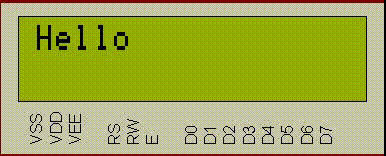# Print ASCII characters on LCD 16×2 using Arduino

Before print ASCII characters on LCD 16×2 using Arduino, you must know about ASCII, ASCII stands for AMERICAN STANDARD CODE FOR INFORMATION INTERCHANGE.

The ASCII table has 128 characters, ranging from 0 to 127. In this manner, 7 bits are sufficient to represent a character in ASCII (27 = 128).

## ASCII TABLE

In the table, we can see Decimal and Hexadecimal values for each character, Decimal values from 0 to 31 and 127, are non-printable control characters. Every single other character can be printed by the PC, i.e. shown on the screen are called printable characters.

## PRINTING CONCEPT

Every character have an ASCII number
97 for ‘a’
65 for ‘A’
49 for ‘1′
35 for ‘#’ etc.

The concept is so simple; each character variable holds ASCII value (an integer number between 0 and 127) rather than the character itself. Means for ‘a’ it will store 97 and so on.

We can take an input from the user in integer form or character form. So we can print it in both forms

1. In character form – It will print character corresponding to ASCII number
2. In integer form – It will print ASCII number corresponding to the character

As we want to print character at different positions in ASCII table, it may be the character whose ASCII value maybe 65 or 91, for this we give printing command to “character variable s” but that character variable contain integer number, So the character corresponding to an integer value in ASCII table will be print. The example below will make it more clear.

For this, we define character variable “s” & in for loop under void loop () function, assigning integer (that is i) values from 33 to 127 to “s” and print them one by one in character form (Here we are printing characters for the numbers from 35 to 127).

You might be thinking of why we are printing characters from 33 to 127 so your guess is correct because ASCII numbers from 0 to 32 are printable.

## CONNECTION OF LCD 16X2 AND ARDUINO

S.N.Arduino PinLCD pin
1.1. VSSGround (0V)
2.2. VCC+ 5V
3.3. VEE10K POT
4.24. RS
5.5. RWGround
6.36. E
7.7. D0-
8.8. D1-
9.9. D2-
10.10. D3-
11.411. D4
12.512. D5
13.613. D6
14.714. D7
15.15. Anode ++ 5V
16.16. Cathode -0V (Ground)

### CIRCUIT DIAGRAM* POT = Potentiometer = Variable resistor

## ARDUINO CODE TO PRINT ASCII CHARACTERS ON LCD 16X2 USING ARDUINO

```#include<LiquidCrystal.h>
LiquidCrystal lcd(2, 3, 4, 5, 6, 7);
char s;
int i;

void setup() {
lcd.begin(16, 2);
lcd.print("Hello");
delay(1000);
lcd.clear();
}

void loop() {
for (i = 33 ; i <= 127 ; i++)  {
s = i;
lcd.setCursor(0, 0);
lcd.print(s);
delay(400);
}
// to not repeat the loop again and again
while (1);
}```

There is also an extended version of ASCII available from 128 to 255 which uses 8 bit instead of 7 bit which gives us extra 128 characters, try this at your own and see how those ASCII characters look like.

#### OUTPUT